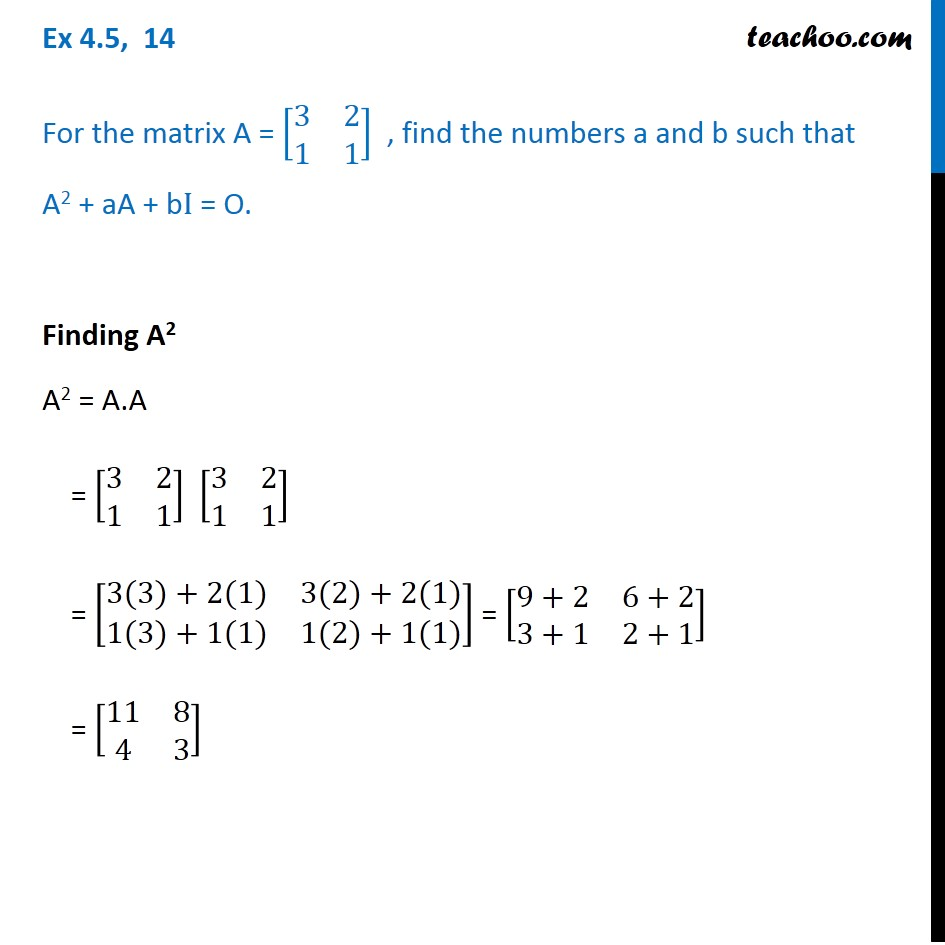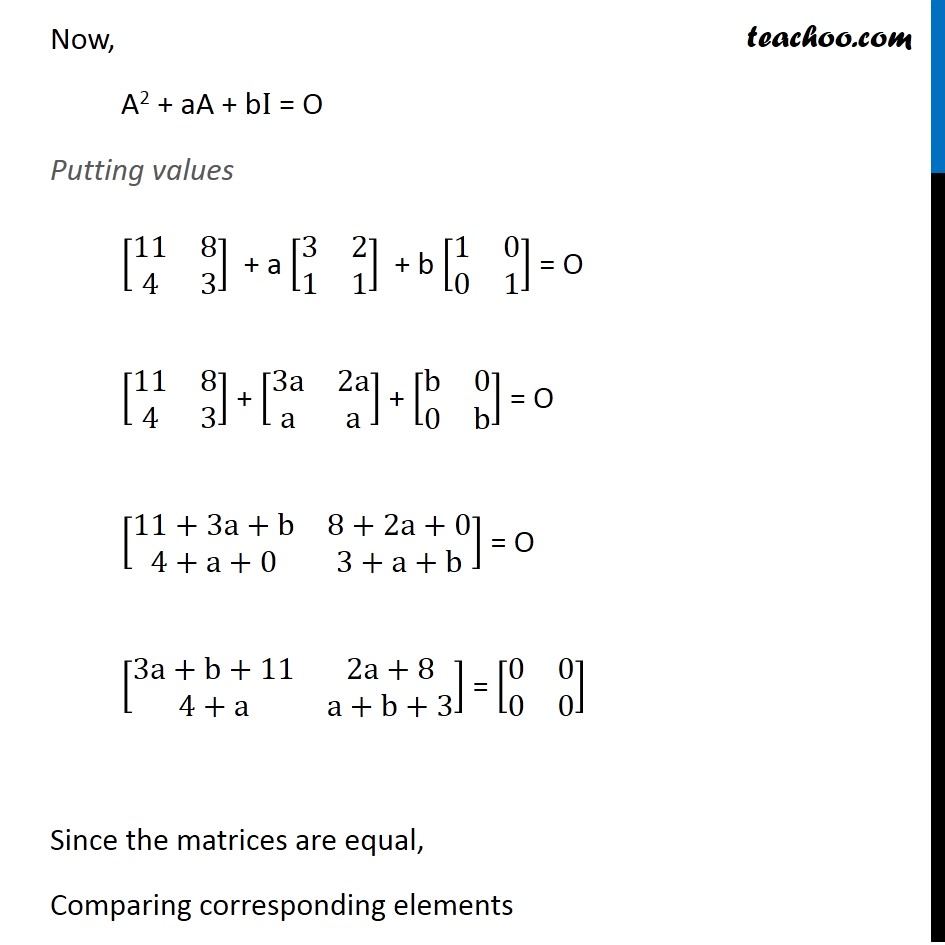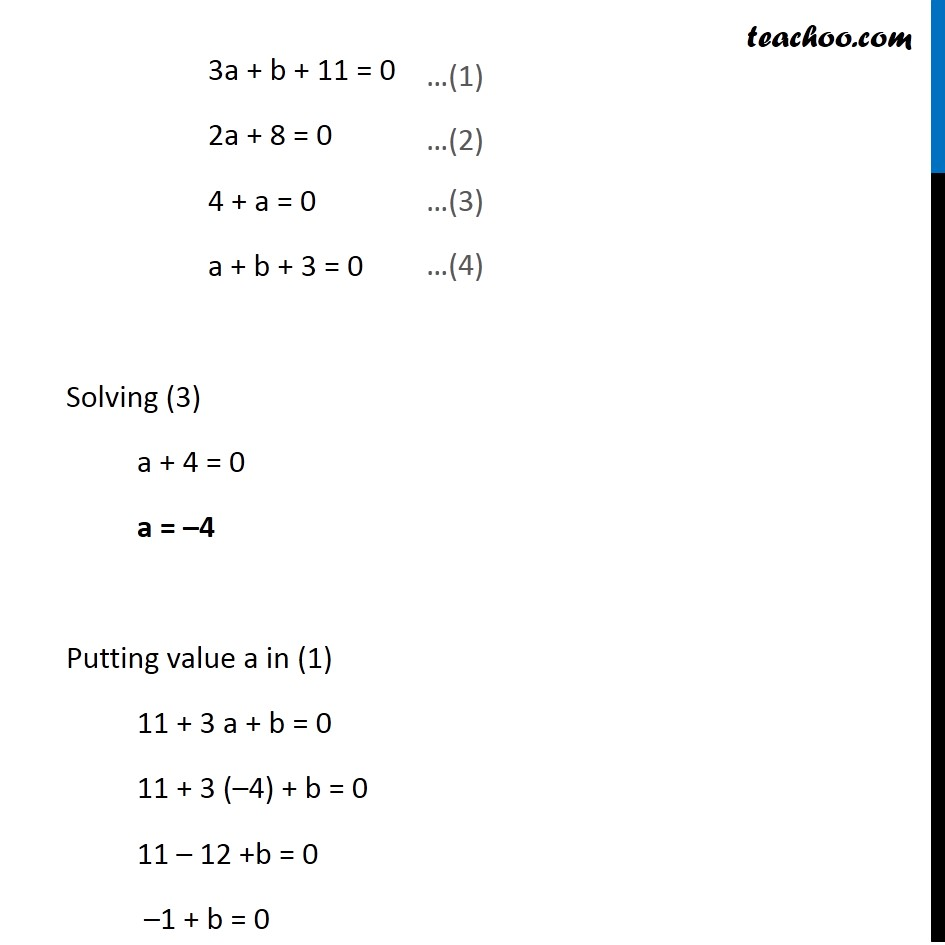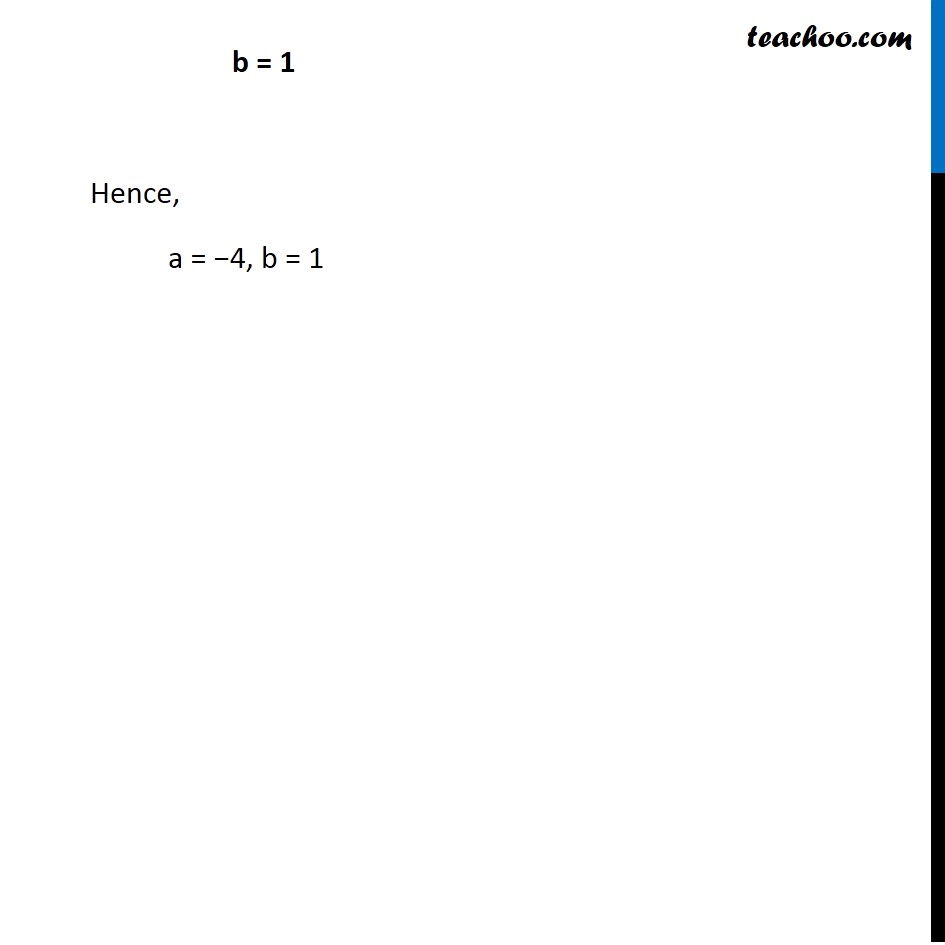Finding inverse when Equation of matrice given

Chapter 4 Class 12 Determinants
Concept wiseIntroducing your new favourite teacher - Teachoo Black, at only ₹83 per month

### Transcript

Ex 4.5, 14 For the matrix A = [■8(3&2@1&1)] , find the numbers a and b such that A2 + aA + bI = O. Finding A2 A2 = A.A = [■8(3&2@1&1)] [■8(3&2@1&1)] = [■8(3(3)+2(1)&3(2)+2(1)@1(3)+1(1)&1(2)+1(1))] = [■8(9+2&6+2@3+1&2+1)] = [■8(11&8@4&3)] Now, A2 + aA + bI = O Putting values [■8(11&8@4&3)] + a [■8(3&2@1&1)] + b [■8(1&0@0&1)] = O [■8(11&8@4&3)] + [■8(3a&2a@a&a)] + [■8(b&0@0&b)] = O [■8(11+3a+b&8+2a+0@4+a+0&3+a+b)] = O [■8(3a+b+11&2a+8@4+a&a+b+3)] = [■8(0&0@0&0)] Since the matrices are equal, Comparing corresponding elements 3a + b + 11 = 0 2a + 8 = 0 4 + a = 0 a + b + 3 = 0 Solving (3) a + 4 = 0 a = –4 Putting value a in (1) 11 + 3 a + b = 0 11 + 3 (–4) + b = 0 11 – 12 +b = 0 –1 + b = 0 …(1) b = 1 Hence, a = −4, b = 1